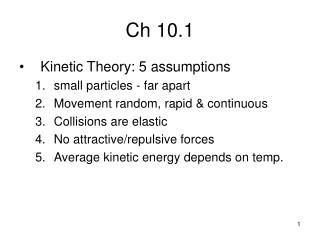DownloadDownload PresentationCh 10.1

# Ch 10.1

Download Presentation## Ch 10.1

- - - - - - - - - - - - - - - - - - - - - - - - - - - E N D - - - - - - - - - - - - - - - - - - - - - - - - - - -
##### Presentation Transcript

1. Ch 10.1 • Kinetic Theory: 5 assumptions • small particles - far apart • Movement random, rapid & continuous • Collisions are elastic • No attractive/repulsive forces • Average kinetic energy depends on temp.

2. Ch 10.1 • Properties specific to gases: • Expand • Compress • Low density • Fluid • Diffuse: [↑]to [↓] until equal • Rate depends on, speed, diameter, attractive force • Calculated by v1m2 • v2 m1 • Effuse: force through small opening. • Joule Thomas effect

3. Ch 10.2 • Note: the lower the temp of a gas the more “ideal” it is • Pressure = caused by the collisions of gas particles against it’s container. • Standard Pressure units • 101.3 kPa= • 760mmHg or Torr for Torricilli (barometer) • 1 ATM • STP = standard temp and pressure

4. Ch 10.2 • Standard Temperature • 0 oC or 273 K • Temp is a measure of kinetic energy • Kinetic energy = mv2 / 2 • Absolute zero is where (theoretically) all particle motion ceases.-273 oC or 0 K

5. Ch 10.3 • In 1662 Robert Boyle: If amount and temp remain the same then, • Boyle’s Law = P1V1 = P2V2 • Inverse proportion: As one goes up the other goes down.

6. Ch 10.3 • 1787 Jacques Charles showed that the volume of a gas varied directly with Kelvin temp • Charles’ Law: V1 = V2 • T1 T2 • K= oC + 273 • *** always calculate in Kelvin for gases***

7. Ch 10.3 • 1802 Joseph Gay-Lussac recognized that for each 1oC increase the volume would increase by 1/273. • Therefore at -273oC a gas would have no volume or disappear BUT ALL gases turn to liquid before this happens. • He also noticed : P1 = P2 • T1 T2

8. Ch 10.3 • The Combined Gas Law: P1V1 = P2V2 T1 T2

9. Ch 10.3 • John Dalton found that in the absence of a chem rxn the pressure of a gas mixture is the sum of the individual gas pressures. • The partial pressure is the pressure of one gas in a mixture so it’s only part of the total pressure! • P total = P gas + P other gases • P gas = P total – P other gases

10. CH 10.3 • When a gas is collected through water displacement –The left over water vapor pressure exerts a pressure that must be accounted for. • Use a water vapor chart (pg 899) • Pgas = Patm – PH2O at what ever temp

11. CH 10.3 • A sample of Oxygen gas is collected in a 175ml container over water at 15oC and the barometer read 760 torr. What volume will the dry gas occupy at 770torr and 15oC. • Adjusting for the water vapor 760 torr - 12.8 torr = 747.2 torr should be used instead of 760 torr.

12. IDEAL GAS Law • Ideal Gas equation is derived at by combining all 4 variables concerned with the physical characteristics of gases (volume, temperature, pressure & # of particles)

13. IDEAL GAS Law • Ideal Gas Equation: PV=nRT • R is a constant used in calculations involving the ideal gas equation • R= .0820 L•Atm • mol•K • R = (1atm)(22.4L)/ (1mol)(273K) • n = number of moles

14. Molecular Mass and Density • The calculation of the molecular mass (M) and Density (D) can be determined using the ideal gas equation. • M = mRT D= MP PV RT This is important in determining the formulas and structures of unknown compounds.